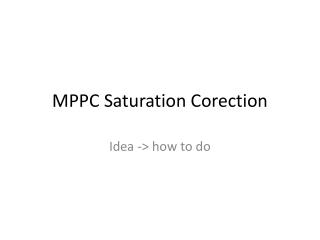DownloadDownload PresentationMPPC Saturation Corection

MPPC Saturation Corection

Download PresentationMPPC Saturation Corection

- - - - - - - - - - - - - - - - - - - - - - - - - - - E N D - - - - - - - - - - - - - - - - - - - - - - - - - - -
Presentation Transcript

1. MPPC Saturation Corection Idea -> how to do

2. MPPC Gain measurement • To apply saturation correction to the beam data, all MPPC are needed to be calibrated from ADC count to number of fired pixels. • Gain of the MPPC has been measured with LED system during no-beam period. There are two modes with readout electronics : • High-gain mode (Calibration mode) used to see 1 p.e peaks for MPPC gain monitoring.LED run data were taken with high gain mode. • Low-gain mode (Physics mode) Have lower gain to enhance ADC upper range, Beam data were taken with this mode. • To utilize d values in high gain mode to the saturation correction for beam data, we have to translate d values from high gain mode to low gain mode by measuring the ratios of gains in two different mode called Inter-calibration factor inter-calibration factor = high gain / low gain • Now we can calculate d-values and its uncertainties in low gain mode dlow gain = dhigh_gain/ Inter.Calib.Factor

3. MPPC response correction AS MPPC has limited number of pixels and one pixel can count only one photon at same . This mean MPPC is not linear to the input light SO MPPC correction is necessary. Theoretically MPPC response curve can be calculated as Nfired : No of pixels which fired signal, Npix : No of pixels on sensor, Np.e : No of photon electrons injected. If Np.e << Nfired correspond to linear response, and if Np.e> or = Nfired, the MPPC response goes to saturate and become flat

4. Correction Procedure • First measure the d values to translate the MPPC output in the beam data from the ADC counts to number of fired pixels to apply saturation correction. • translate the MPPC output from the ADC counts to number of fired pixels, and correct it to the number of photo electrons using correction function. DC / dhigh-gain

5. In the .CC file • Two data file one is for inter-calibration factor i.eInt.calib= dhighgain/dlowgain Layer strip interClibMeaninterCalibMeanError • 1 15.4623 ± 16.2385 1 2 0 ± 15.3931 1 3 14.4794 ± 16.325 1 4 16.9113 ± 16.0809 1 5 15.0477 ±16.1837 • Another is gain calibration file i.ed_values at high gain mode Layer strip gainClibMean gainCalibMeanError • 1 214.199 ± 0.669616 1 2 205.172 ± 0.59258 1 3 209.989 ± 0.63876 1 4 212.075 ± 0.55146 1 5 205.241± 0.555516 After reading the d_values, Temperature correction is also applied to the gain calibration constant (d_vaues) Then the collected hits is converted into number of photon before applying the saturation correction function, ampl= ampl / (d_values / interCalibConst[l][s]) And then the saturation correction function for the number of photon is used. ampld = - effNpix * log(1. – ampl / effNpix) After applying the saturation function we need to convert it again to ADC counts ampl = ampld * (gainClibConst[l][s] / interClibConst[l][s]) And finally mipcalib constant corrected with temperature is used in order to get adc counts in unit osmips

6. My question is • I have two data file for inter calibration constant and gain calibration constant . Do I have to generate 20 files randomly using the same way as I did for MIP and Temp for both data files ? And perform pseudo experiment 20 times for intercalibration and 20 times for gain calibration? And how about saturation factor, do I need to use only minimum and maximum values (1600pix, ?) ?

7. x102 (ADC) Fitting result MPPC response x10 (ADC) PMT response MPPC Saturation Correction • the response curve is fitted using the following function to extract the effective number of pixels, which includes the effect of dynamic range enhancement due to quick recovery of MPPC and this function was used to make saturation correction Response curve for 1600-pixel MPPC Where, Nfired is the number of photon detected by MPPC, ɛ is the photon detection efficiency, Npix is the number of pixels on the MPPC, Nin is the number of photons incident in the MPPC sensitive area Result of fitting: Npix= 2424±3